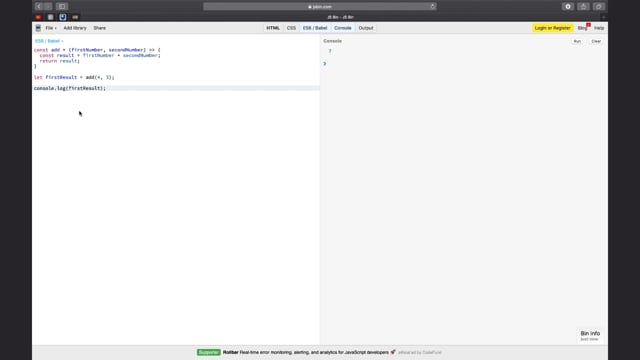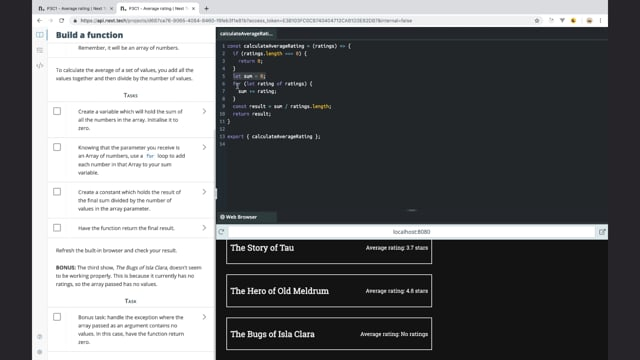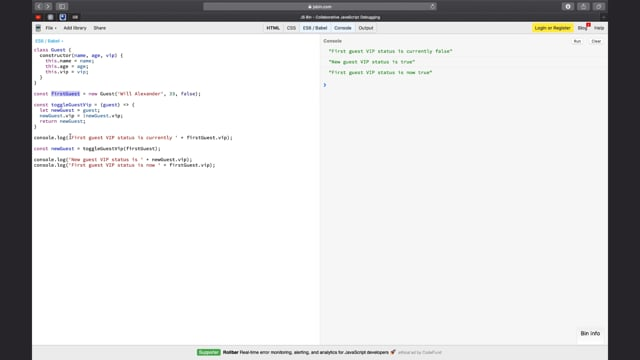Last updated on 5/4/22

## Understand parameters and return valuesA function is a block of code with a specific purpose to which you give a name. When you call that function, you execute the code it contains. For example, in the screencast videos, you've seen me call the  `console.log()`  function, which contains code that prints stuff to the console.

Many functions require variables to do their job. When you create or declare a function, you list the variables it requires to do its job: you set parameters for the function.

Then, when you call the function, you pass it values for those parameters. The values are called arguments.

Finally, your function may give you a result: a return value. Let's say you have a function that counts the number of words in a string:

• The parameter is a string in which you will count the words.

• The argument is any string you pass to your function when you call it.

• The return value is the number of words.#### Practice working with functions!

Your video streaming app allows users to rate shows out of 5 stars. Your colleague has built the component to show the average score for each show, but needs you to write the function that will calculate that average. She explains that the function must take an array of numbers as a parameter and return the average score as a number.

1. In the appropriate place in your function declaration, pick a name for the parameter for your function.
Remember, it will be an array of numbers.
To calculate the average of a set of values, you add all the values together and then divide by the number of values.

2. Create a variable which will hold the sum of all the numbers in the array. Initialize it to zero.

3. Knowing that the parameter you receive is an `array` of numbers, use a for loop to add each number in that `array` to your sum variable.

4. Create a constant which holds the result of the final sum divided by the number of values in the array parameter.

5. Have the function return the final result, then check that it works!

6. BONUS: The third show, The Bugs of Isla Clara, doesn't seem to be working properly. This is because it currently has no ratings, so the array passed has no values. Handle the exception where the array passed as an argument contains no values. In this case, have the function return zero.

#### Solution:

Once you've given it a go, watch me code a solution and see how your approach compares. And here is a new CodePen with a solution to the exercice.In the previous section, we looked at functions which manipulate values. Even in the case of the exercise where the parameter was an array, we didn't actually manipulate the array: we just looked at the values within it.

You can also create functions which manipulate objects and arrays. To see why this might work a little differently, think back to the difference between primitive and reference types. Variables containing numbers, strings, and booleans, contain values, but those assigned to objects and arrays, contain their reference as opposed to the actual values.

This means that when you pass an object to a function, you are not making a copy of it: you are passing the reference to the original object — the same goes for arrays. This is especially important when returning an object.In this chapter, you learned:

• What a function is, how to declare one, and how to call one.

• About parameters, arguments, and return values.

• To write a function which runs through an array of numbers to calculate their average.

• Why it's important to be careful when using objects and arrays in functions.

In the next chapter, we will look at a kind of function which is associated to a class: methods.